## 8.2Aspect ratio

The previous section mentioned cells of a mesh that are longer in one direction than another. The parameter used to describe this phenomenon is aspect ratio, deﬁned as the ratio of the longest to shortest dimensions,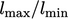, of a cell.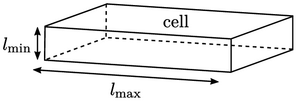Meshes include cells with high aspect ratio for eﬃciency, by exploiting a prior understanding that the gradients in some ﬂow properties e.g. shear stress, deformation rate and velocity, are much greater in one direction than others.

They are most commonly used to resolve the boundary layers at walls. Greater mesh resolution is required normal to the wall, where gradients are particularly high, than tangential to the wall. The centre heights of cells adjacent to the boundary need to be very small, corresponding to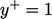.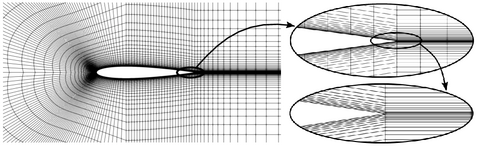In the aerofoil above, the aspect ratio is 1000 at the trailing edge. If those cells were replaced by ones with an aspect ratio of 1, with the same cell height, the number of cells would increase by a factor of 1000, illustrating the extent of the gains in eﬃciency.

Introducing high aspect ratio in one area of the mesh causes problems elsewhere, however. The aerofoil example maintains a high aspect ratio beyond the trailing edge, actually increasing to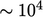further downstream. If the ﬂow deviates from a direction parallel to the long side of the high aspect ratio cells, transient simulations then require very small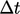to fall within reasonable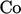limits. The mesh can be modiﬁed by abruptly reducing the aspect ratio beyond the trailing edge, but this increases the error associated with discretisation.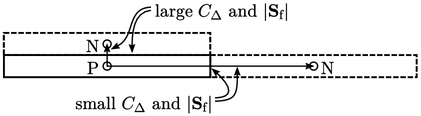With Laplacian discretisation by Eq. (3.2 ) and Eq. (3.5 ), the matrix coeﬃcients include a factor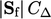. On a regular mesh, both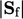and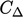are larger for the faces across the longest side of a high aspect ratio cell, than the shortest side, by a factor equal to the aspect ratio. Matrix coeﬃcients can thereby diﬀer by a factor of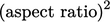.

The convergence of the pressure equation, dominated by the Laplacian derivative, is adversely aﬀected, in particular with the CG-based matrix solvers. The GAMG solver fares much better because the agglomeration strategy is based on a progressive reduction in aspect ratio as discussed in Sec. 5.18 .

A matrix with a wide range of coeﬃcient values yields lower residual values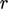, calculated from Eq. (5.11 ). In other words, for a given, a solution with high aspect ratio cells is less converged than one without. Therefore, to maintain an equivalent level of convergence with high aspect ratio cells, tolerance controls need to be reduced, often by a few orders of magnitude.

Notes on CFD: General Principles - 8.2 Aspect ratio